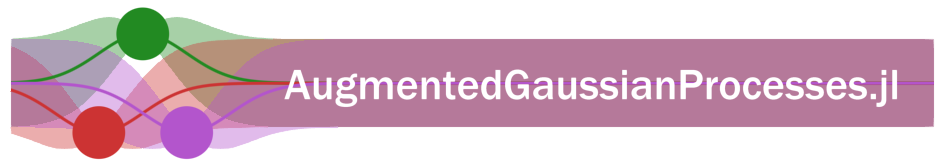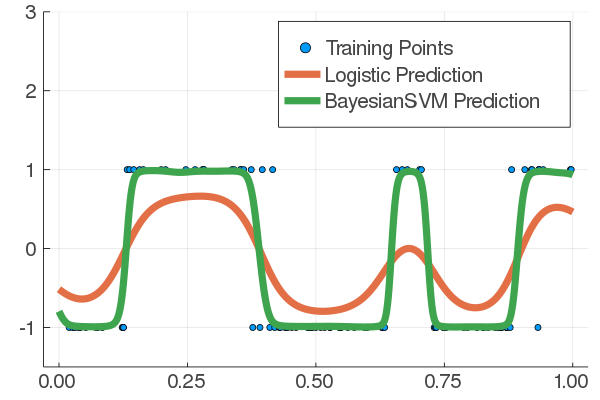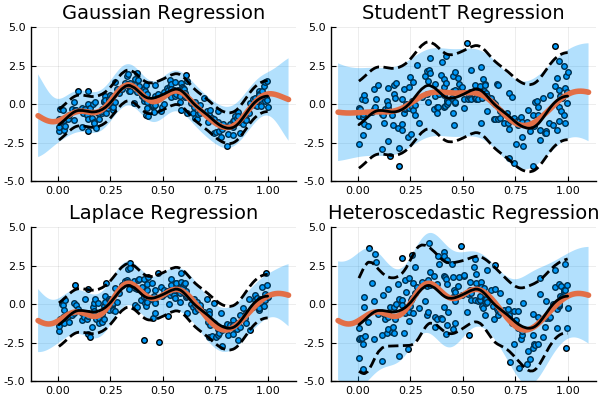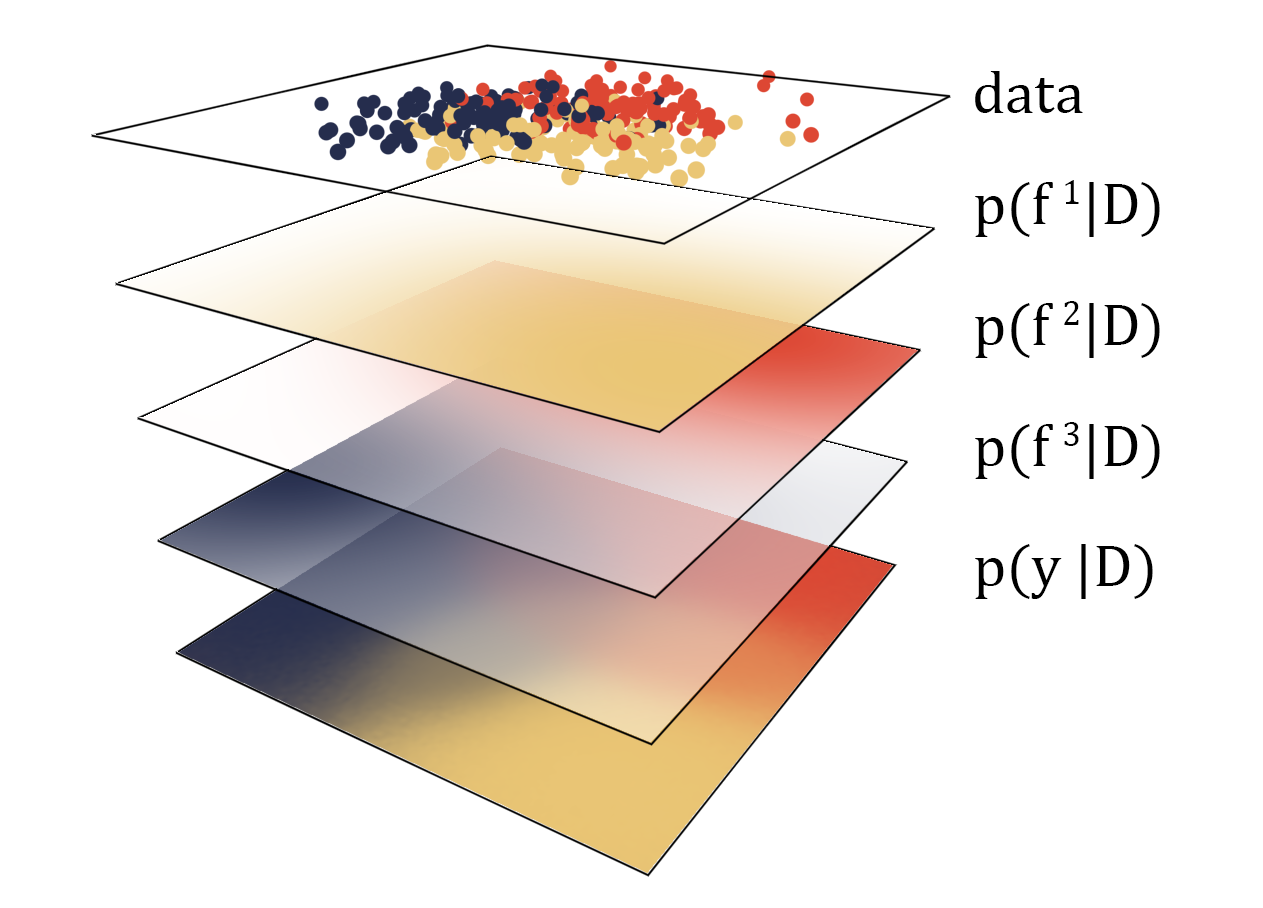AugmentedGaussianProcesses

44

7

3

3

contributorsAugmentedGaussianProcesses.jl is a Julia package in development for Data Augmented Sparse Gaussian Processes. It contains a collection of models for different gaussian and non-gaussian likelihoods, which are transformed via data augmentation into conditionally conjugate likelihood allowing for extremely fast inference via block coordinate updates. There are also more options to use more traditional variational inference via quadrature or Monte Carlo integration.

Packages models :

Two GP classification likelihoodFour GP Regression likelihood

• Gaussian : The standard Gaussian Process regression model with a Gaussian Likelihood (no data augmentation was needed here) IJulia example/Reference
• StudentT : The standard Gaussian Process regression with a Student-t likelihood (the degree of freedom ν is not optimizable for the moment) IJulia example/Reference
• Laplace : Gaussian Process regression with a Laplace likelihood IJulia example/(No reference at the moment)
• Heteroscedastic : Regression with non-stationary noise, given by an additional GP. (no reference at the moment)One Multi-Class Classification Likelihood

• Logistic-SoftMax : A modified version of the softmax where the exponential is replaced by the logistic function IJulia example/ReferenceMore models in development

• Poisson : For point process estimation
• Probit : A Classifier with a Bernoulli likelihood with the probit link
• Online : Allowing for all algorithms to work online as well

Install the package

The package requires at least Julia 1.1 Run julia, press ] and type add AugmentedGaussianProcesses, it will install the package and all its dependencies.

Use the package

A complete documentation is available in the docs. For a short start now you can use this very basic example where X_train is a matrix N x D where N is the number of training points and D is the number of dimensions and Y_train is a vector of outputs (or matrix of independent outputs).

using AugmentedGaussianProcesses
model = SVGP(X_train,Y_train,RBFKernel(1.0),LogisticLikelihood(),AnalyticSVI(100),64)
train!(model,iterations=100)
Y_predic = predict_y(model,X_test) #For getting the label directly
Y_predic_prob, Y_predic_prob_var = proba_y(model,X_test) #For getting the likelihood (and likelihood uncertainty) of predicting class 1

Both documentation and examples/tutorials are available.

References :

Check out my website for more news

"Gaussian Processes for Machine Learning" by Carl Edward Rasmussen and Christopher K.I. Williams

UAI 19' "Multi-Class Gaussian Process Classification Made Conjugate: Efficient Inference via Data Augmentation" by Théo Galy-Fajou, Florian Wenzel, Christian Donner and Manfred Opper https://arxiv.org/abs/1905.09670

ECML 17' "Bayesian Nonlinear Support Vector Machines for Big Data" by Florian Wenzel, Théo Galy-Fajou, Matthäus Deutsch and Marius Kloft. https://arxiv.org/abs/1707.05532

AAAI 19' "Efficient Gaussian Process Classification using Polya-Gamma Variables" by Florian Wenzel, Théo Galy-Fajou, Christian Donner, Marius Kloft and Manfred Opper. https://arxiv.org/abs/1802.06383

UAI 13' "Gaussian Process for Big Data" by James Hensman, Nicolo Fusi and Neil D. Lawrence https://arxiv.org/abs/1309.6835

JMLR 11' "Robust Gaussian process regression with a Student-t likelihood." by Jylänki Pasi, Jarno Vanhatalo, and Aki Vehtari. http://www.jmlr.org/papers/v12/jylanki11a.html

01/25/2018

1 day ago

468 commits